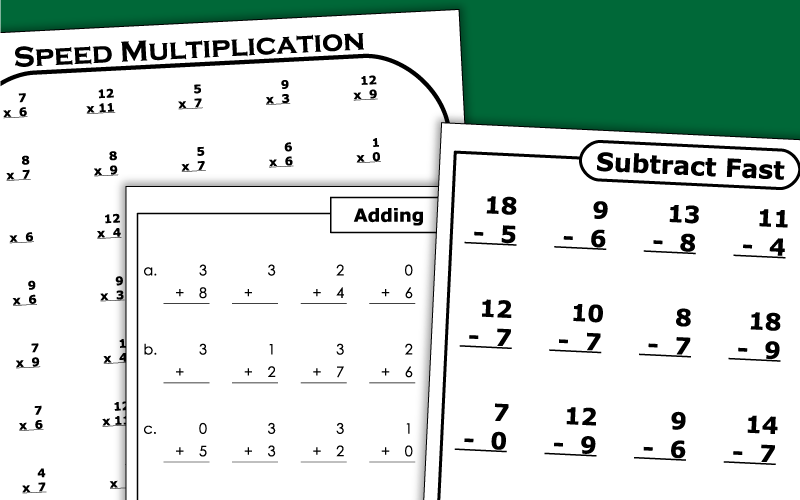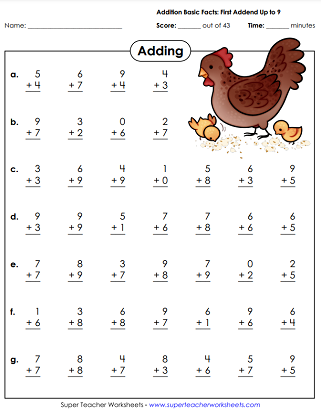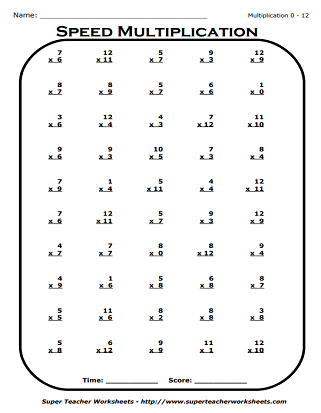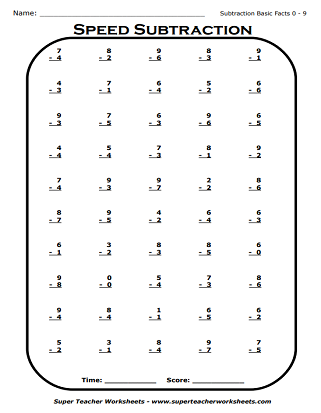# Minute Math Drills (Mad Minute Drills)

Minute Math Drills, or Math Mad Minutes as they are known to many teachers, are worksheets with simple drill-and-practice basic facts math problems.  Students are given a short period of time (usually three minutes or so) to complete as many problems as they can.  When done on a regular basis, these timed worksheets will help students improve speed and accuracy with basic adding, subtracting, multiplication, and division facts.This practice worksheet has pictures of kid-friendly monsters. The first addend for each problem is 0, 1, or 2.  The second addend is 0 through 9.
Practice forty-three addition facts on this worksheet.  The first addend is between 0 and 3.  Worksheet has roller coaster graphics.
Another basic addition drill worksheet.  This time, the first addend is between 0 and 4.  Horse graphics decorate this worksheet.
The first addend for each of the problems on this worksheet is between 0 and 5.  Worksheet has pictures of playful monkeys.
Basic facts drill worksheet in which the first addend ranges from 0 to 6.  Worksheet features race car graphics.
The first addend for each fact on this printable is between 0 and 7.  Features adorable kitty pictures.
Even more basic addition facts.  This time, the first addend for each problem is between 0 and 8.  Cute wizard pictures.
Basic addition worksheet.  First addend is between 0 and 9.  Includes cartoon frog pictures.

## Subtraction Basic Facts (0-18) Mad Minute Drills

How many of these 25 subtraction facts (0-18) can your students complete in one minute?
Looking for more than 25 facts?  Try this worksheet.  It has 50!

## Division Basic Facts Mad Minute Drills

Students must write the quotients of these basic division problems.  Problems have divisors up to 12.

## Also on Super Teacher Worksheets

Practice higher-level addition, with and without regrouping.

Division Worksheets

Basic division fact worksheets and higher-level division.

Multiplication Worksheets

Tons of basic fact practice activities, as well as higher levels of multiplication

Subtraction Worksheets

Subtraction of 1, 2, 3, and 4-digit numbers, with and without regrouping.

## Sample Worksheet ImagesMy Account
Site Information